# DIFFERENTIATION Outcome 3 Using differentiation Application Finding the

• Slides: 93DIFFERENTIATION Outcome 3 Using differentiation (Application) Finding the gradient for a polynomial Increasing / Decreasing functions Differentiating Brackets ( Type 1 ) Max / Min and inflexion Points Differentiating Harder Terms (Type 2) Curve Sketching Differentiating with Leibniz Notation Max & Min Values on closed Intervals Equation of a Tangent Line ( Type 3 ) Optimization Mind Map of ChapterGradients & Curves Outcome 3 Higher On a straight line the gradient remains constant, however with curves the gradient changes continually, and the gradient at any point is in fact the same as the gradient of the tangent at that point. S B The sides of the half-pipe are very steep(S) but it is not very steep near the base(B). Demo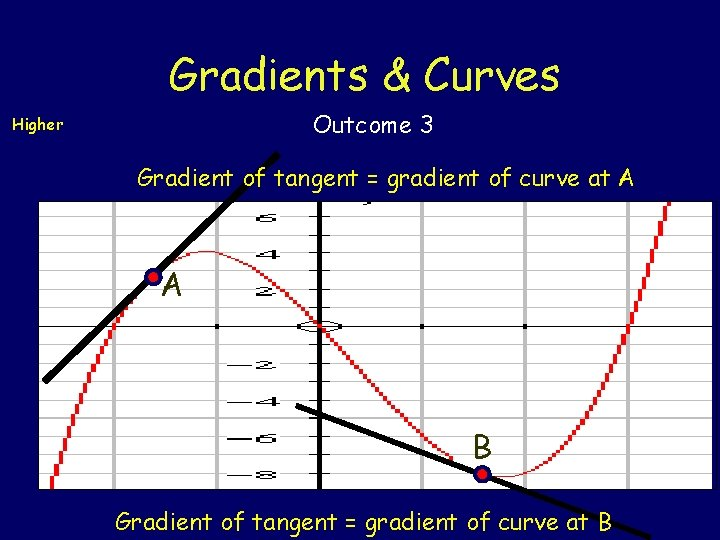To find the gradient at any point on a curve we need to modify the gradient formula Gradients & Curves Higher Outcome 3 For the function y = f(x) we do this by taking the point (x, f(x)) and another “very close point” ((x+h), f(x+h)). Then we find the gradient between the two. ((x+h), f(x+h)) Approx gradient (x, f(x)) True gradient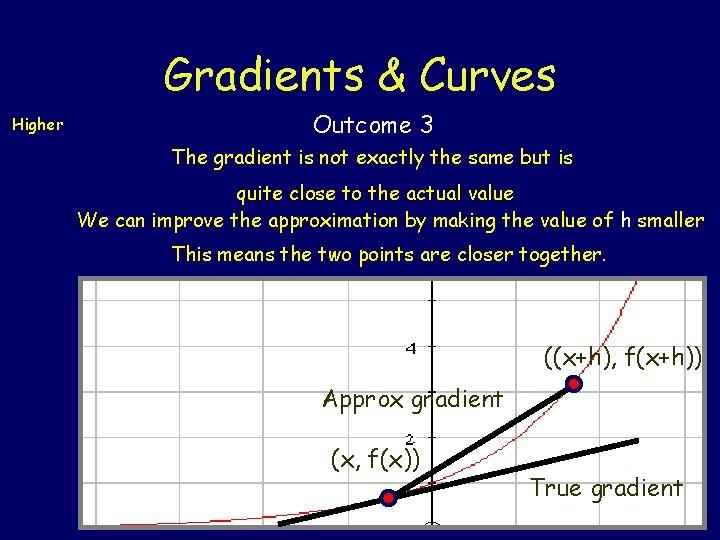Gradients & Curves Higher Outcome 3 The gradient is not exactly the same but is quite close to the actual value We can improve the approximation by making the value of h smaller This means the two points are closer together. ((x+h), f(x+h)) Approx gradient (x, f(x)) True gradientGradients & Curves Higher Outcome 3 We can improve upon this approximation by making the value of h even smaller. So the points are even closer together. Approx gradient (x, f(x)) ((x+h), f(x+h)) True gradient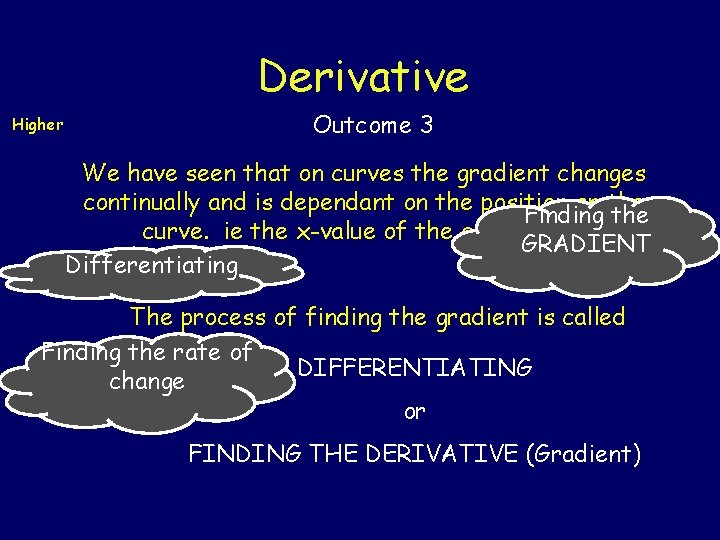Derivative Higher Outcome 3 We have seen that on curves the gradient changes continually and is dependant on the position on the Finding the curve. ie the x-value of the given point. GRADIENT Differentiating The process of finding the gradient is called Finding the rate of DIFFERENTIATING change or FINDING THE DERIVATIVE (Gradient)Derivative Outcome 3 Higher If the formula/equation of the curve is given by f(x) Then the derivative is called f '(x) - “f dash x” f(x) f '(x) There is a simple way 2 x 2 4 x 2 5 x 10 6 x 7 x 3 x 5 x 99 4 x 8 x 50 x 9 42 x 6 3 x 2 5 x 4 99 x 98 of finding f '(x) from f(x). Have guessed the rule yet !Derivative Higher Rule for Differentiating Outcome 3 It can be given by this simple flow diagram. . . multiply by the power If reduce the power by 1 f(x) then f '(x) = axn n 1 = ax NB: the following terms & expressions mean the same GRADIENT, DERIVATIVE, RATE OF CHANGE, f '(x)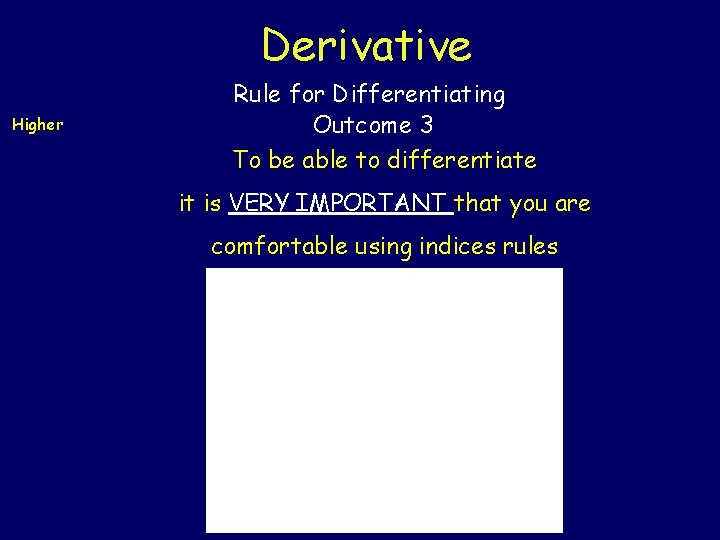Derivative Higher Rule for Differentiating Outcome 3 To be able to differentiate it is VERY IMPORTANT that you are comfortable using indices rules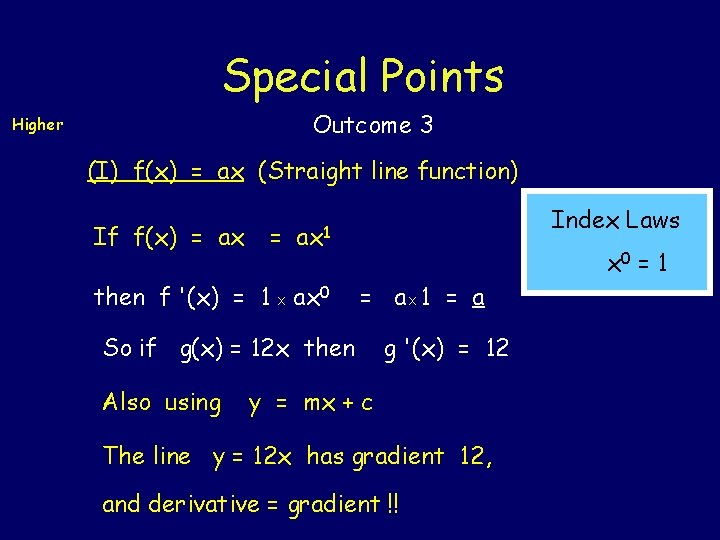Special Points Outcome 3 Higher (I) f(x) = ax (Straight line function) If f(x) = ax = Index Laws ax 1 then f '(x) = 1 X ax 0 = 1 = a. X 1 = a So if g(x) = 12 x then Also using g '(x) = 12 y = mx + c The line y = 12 x has gradient 12, and derivative = gradient !!Special Points Outcome 3 Higher (II) f(x) = a, (Horizontal Line) If f(x) = a X 1 = ax 0 then f '(x) = 0 X ax-1 So if g(x) = -2 then Also using formula Index Laws x 0 = 1 = 0 g '(x) = 0 y = c , (see outcome 1 !) The line y = -2 is horizontal so has gradient 0 !ion t a i nt e i r = e d f Gra Dif Name : Differentiation techniques ge ion n t a a i ch = f ent r o e e f Rat Dif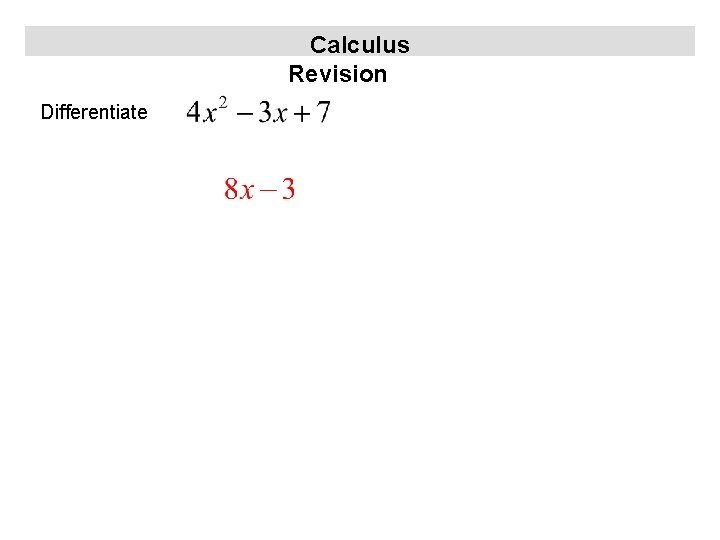Calculus Revision DifferentiateCalculus Revision Differentiate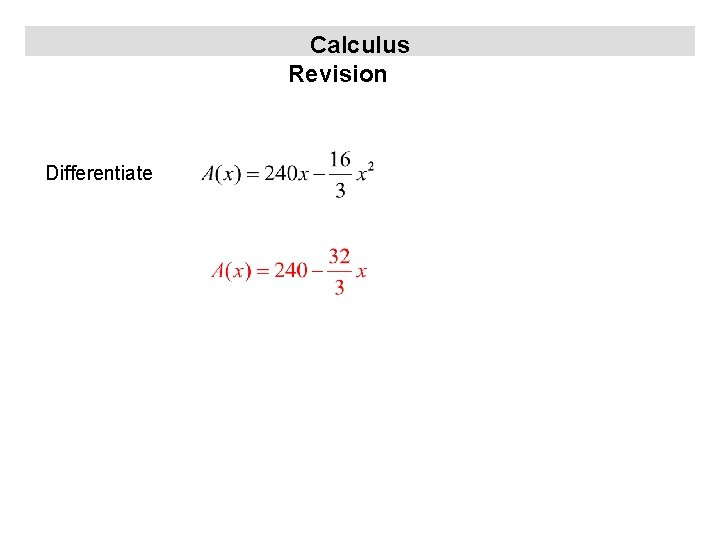Calculus Revision Differentiate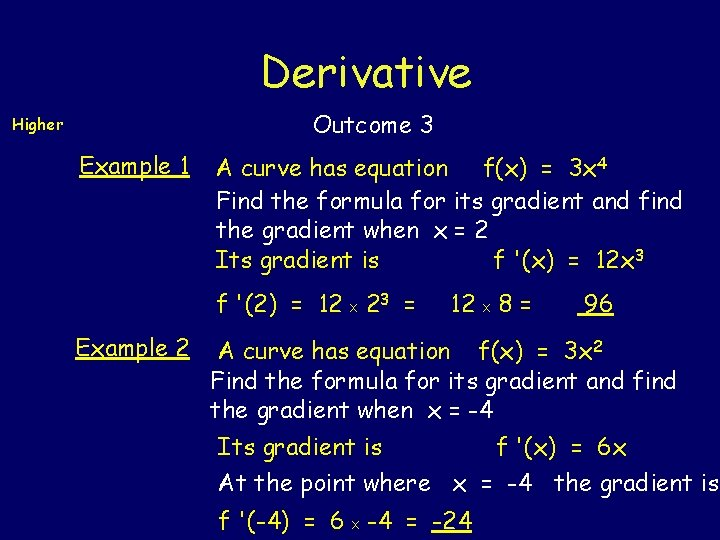Derivative Outcome 3 Higher Example 1 A curve has equation f(x) = 3 x 4 Find the formula for its gradient and find the gradient when x = 2 Its gradient is f '(x) = 12 x 3 f '(2) = 12 X 23 = Example 2 12 X 8 = 96 A curve has equation f(x) = 3 x 2 Find the formula for its gradient and find the gradient when x = -4 Its gradient is f '(x) = 6 x At the point where x = -4 the gradient is f '(-4) = 6 X -4 = -24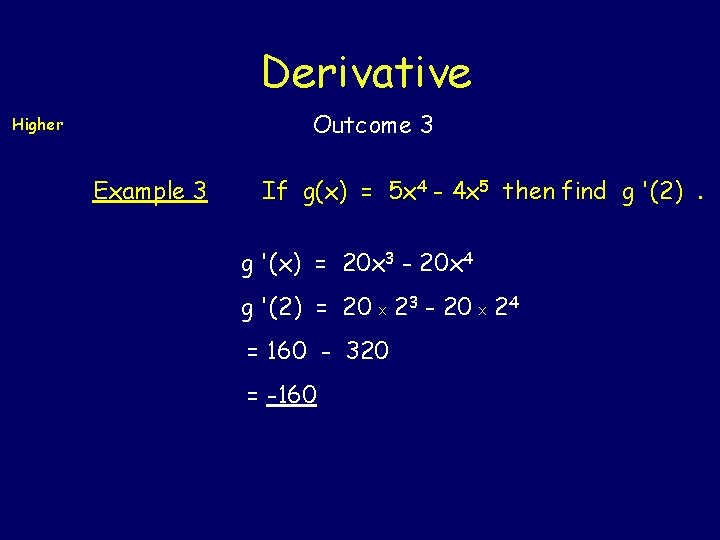Derivative Outcome 3 Higher Example 3 If g(x) = 5 x 4 - 4 x 5 then find g '(2). g '(x) = 20 x 3 - 20 x 4 g '(2) = 20 X 23 - 20 X 24 = 160 - 320 = -160Derivative Outcome 3 Higher Example 4 h(x) = 5 x 2 - 3 x + 19 and so h '(-4) = 10 X (-4) - 3 h '(x) = 10 x - 3 = -40 - 3 = -43 Example 5 k(x) = 5 x 4 - 2 x 3 + 19 x - 8, find k '(10). k '(x) = 20 x 3 - 6 x 2 + 19 So k '(10) = 20 X 1000 - 6 X 100 + 19 = 19419Derivative Outcome 3 Higher Example 6 : Find the points on the curve f(x) = x 3 - 3 x 2 + 2 x + 7 where the gradient is 2. NB: gradient = derivative = f '(x) We need ie f '(x) = 2 3 x 2 - 6 x + 2 = 2 or 3 x 2 - 6 x = 0 ie 3 x(x - 2) = 0 ie so 3 x = 0 or x-2=0 x = 0 or x = 2 Now using original formula f(0) = 7 f(2) = 8 -12 + 4 + 7 =7 Points are (0, 7) & (2, 7)Calculus Revision DifferentiateCalculus Revision Differentiate Straight line form Differentiate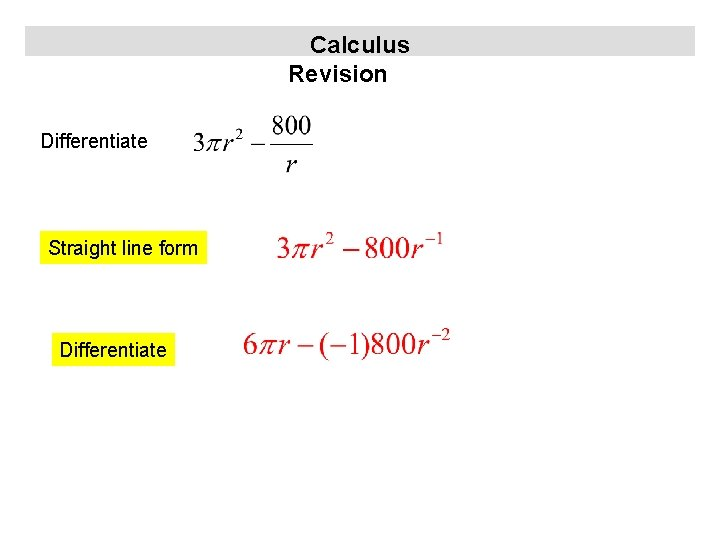Calculus Revision Differentiate Straight line form DifferentiateCalculus Revision Differentiate Straight line form Chain Rule Simplify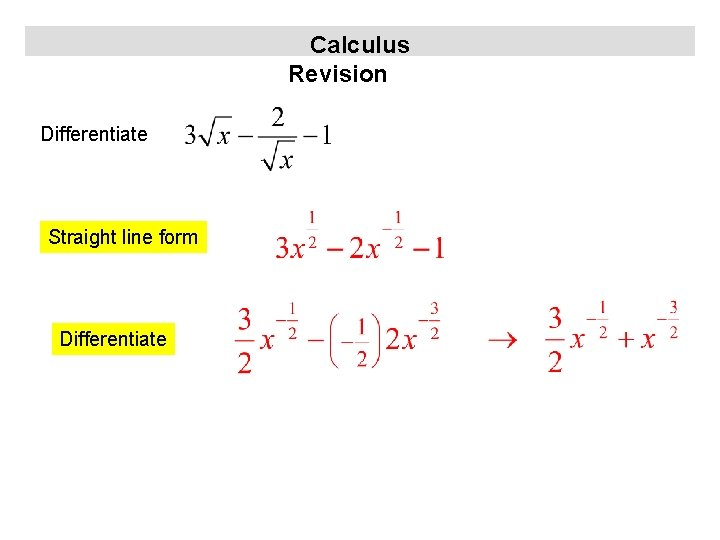Calculus Revision Differentiate Straight line form DifferentiateCalculus Revision Differentiate Straight line form DifferentiateCalculus Revision Differentiate Straight line form Differentiate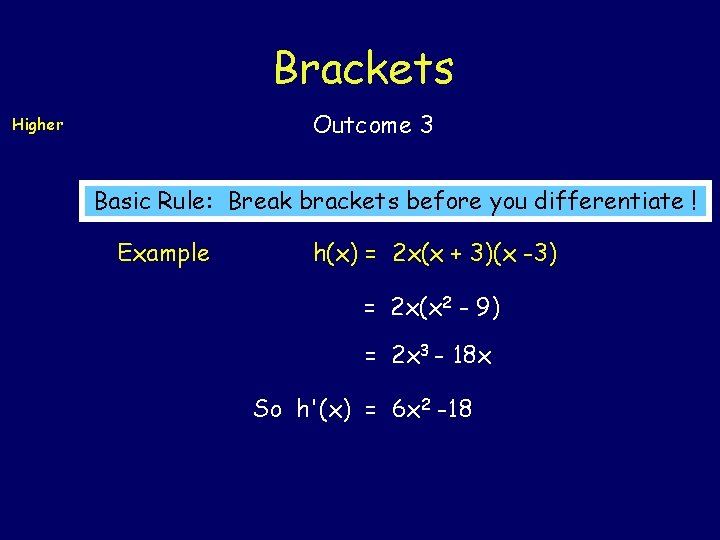Brackets Outcome 3 Higher Basic Rule: Break brackets before you differentiate ! Example h(x) = 2 x(x + 3)(x -3) = 2 x(x 2 - 9) = 2 x 3 - 18 x So h'(x) = 6 x 2 -18Calculus Revision Differentiate Multiply out DifferentiateCalculus Revision Differentiate multiply out differentiate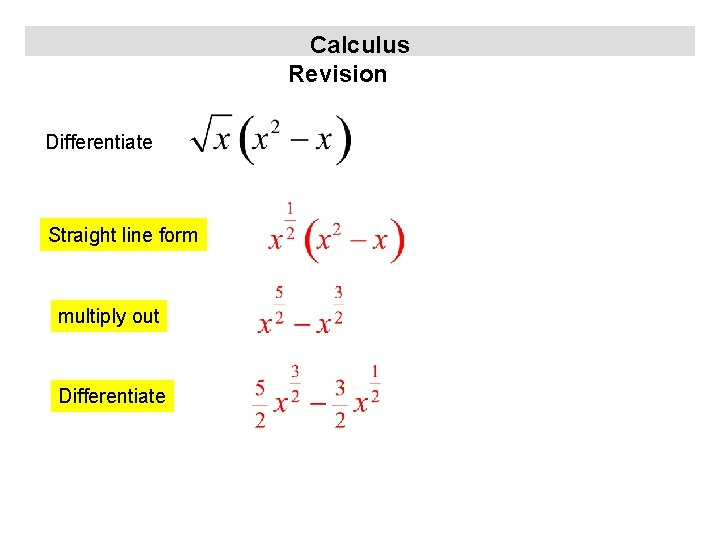Calculus Revision Differentiate Straight line form multiply out DifferentiateCalculus Revision Differentiate multiply out Differentiate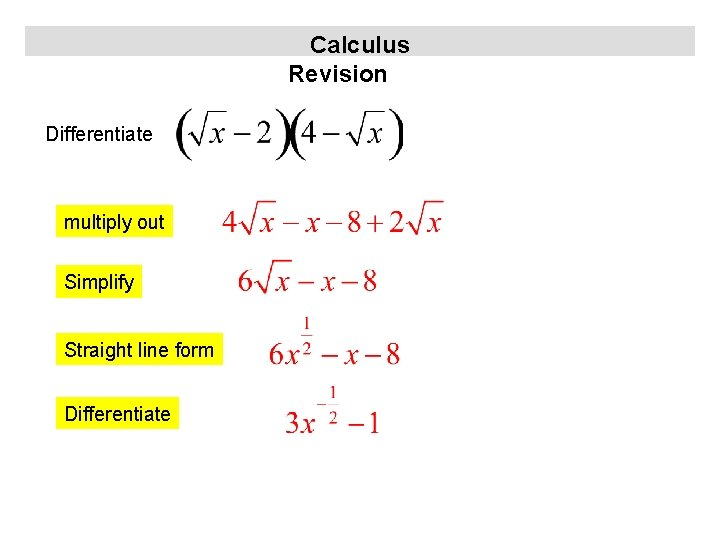Calculus Revision Differentiate multiply out Simplify Straight line form DifferentiateCalculus Revision Differentiate Multiply out Straight line form Differentiate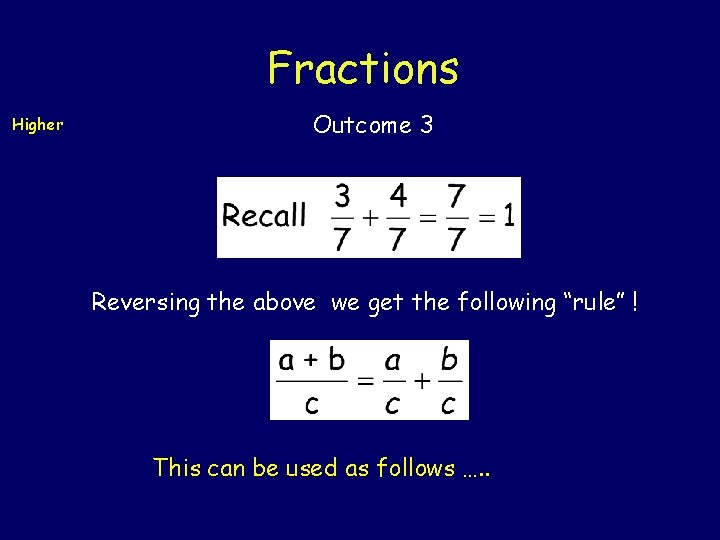Fractions Higher Outcome 3 Reversing the above we get the following “rule” ! This can be used as follows …. .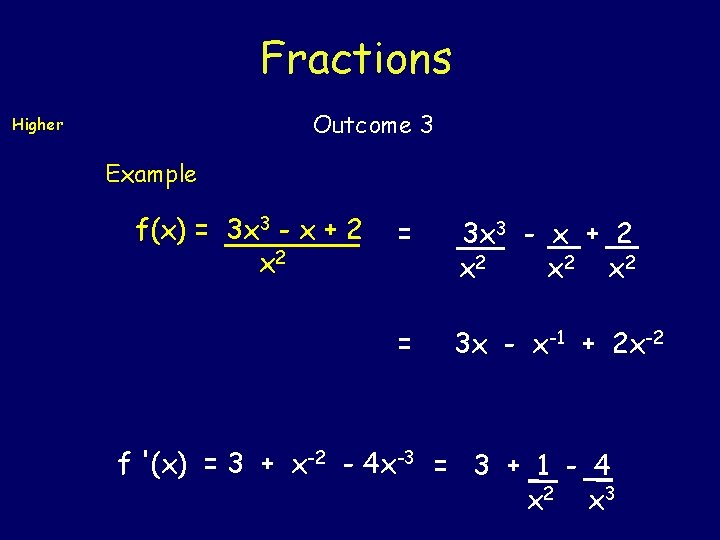Fractions Outcome 3 Higher Example f(x) = 3 x 3 - x + 2 x 2 x 2 = 3 x - x-1 + 2 x-2 f '(x) = 3 + x-2 - 4 x-3 = 3 + 1 - 4 x 2 x 3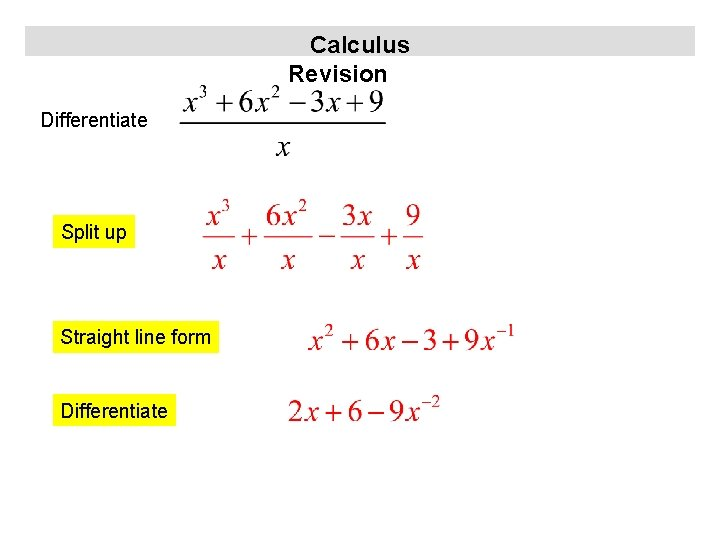Calculus Revision Differentiate Split up Straight line form Differentiate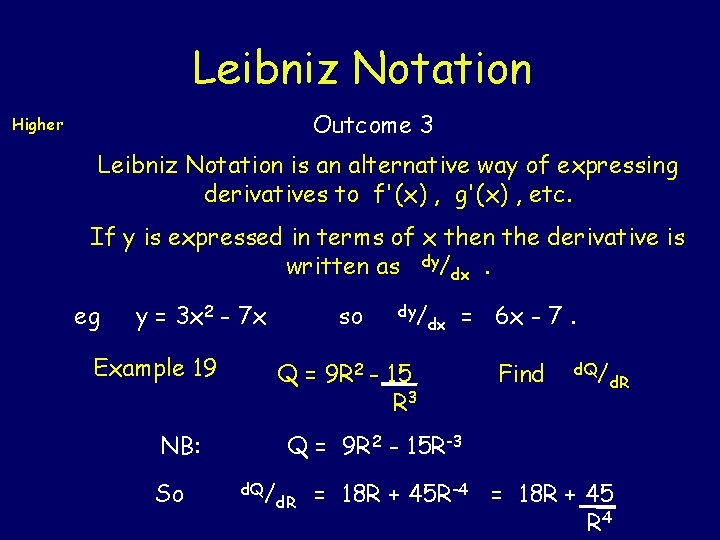Leibniz Notation Outcome 3 Higher Leibniz Notation is an alternative way of expressing derivatives to f'(x) , g'(x) , etc. If y is expressed in terms of x then the derivative is written as dy/dx. eg y = 3 x 2 - 7 x Example 19 dy/ dx = 6 x - 7. Q = 9 R 2 - 15 R 3 NB: So so Find d. Q/ d. R Q = 9 R 2 - 15 R-3 d. Q/ d. R = 18 R + 45 R-4 = 18 R + 45 R 4Leibniz Notation Outcome 3 Higher Example 20 A curve has equation y = 5 x 3 - 4 x 2 + 7. Find the gradient where x = -2 ( differentiate ! ) gradient = dy/ dx = 15 x 2 - 8 x if x = -2 then gradient = 15 X (-2)2 - 8 X (-2) = 60 - (-16) = 76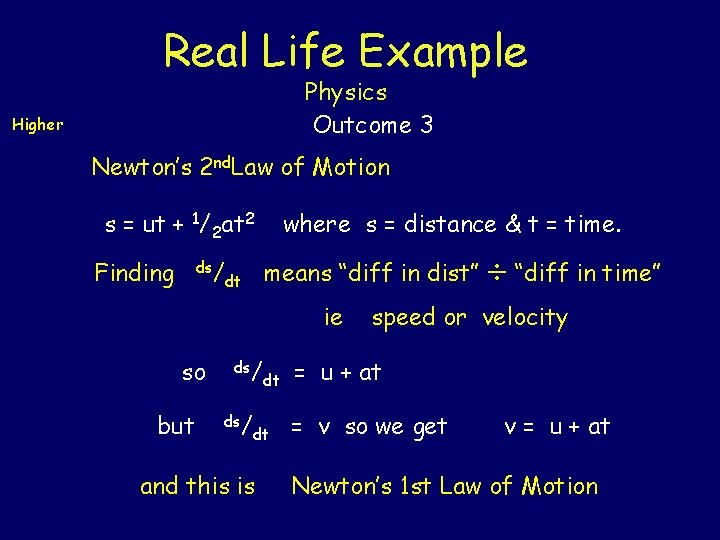Real Life Example Physics Outcome 3 Higher Newton’s 2 nd. Law of Motion s = ut + 1/2 at 2 Finding ds/ where s = distance & t = time. means “diff in dist” dt ie so but ds/ dt dt and this is “diff in time” speed or velocity = u + at = v so we get v = u + at Newton’s 1 st Law of MotionEquation of Tangents y = mx +c Outcome 3 Higher A(a, b) y = f(x) tangent NB: at A(a, b) gradient of line = gradient of curve gradient of line = m (from y = mx + c ) gradient of curve at (a, b) = f (a) it follows that m = f (a)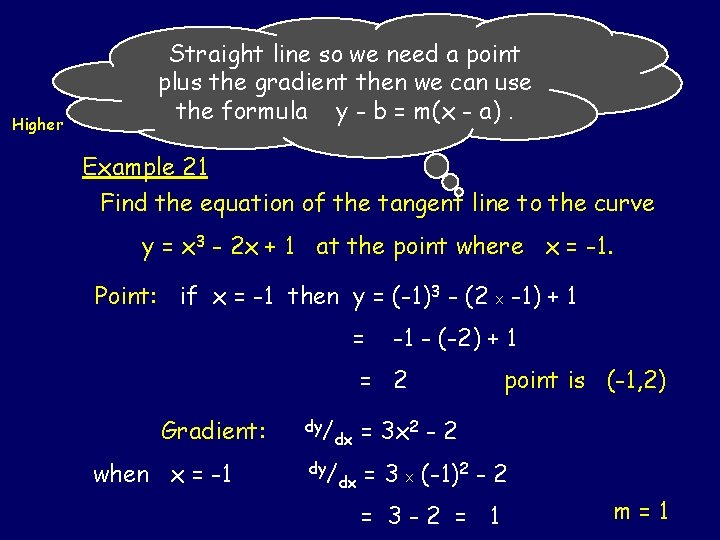Straight line so need a point Equation ofwe. Tangents Higher plus the gradient then we can use the formula y - b = m(x - a). Outcome 3 Example 21 Find the equation of the tangent line to the curve y = x 3 - 2 x + 1 at the point where x = -1. Point: if x = -1 then y = (-1)3 - (2 X -1) + 1 = -1 - (-2) + 1 = 2 Gradient: when x = -1 dy/ dx point is (-1, 2) = 3 x 2 - 2 = 3 X (-1)2 - 2 = 3 -2 = 1 m=1Equation of Tangents Outcome 3 Higher Now using y - b = m(x - a) we get y - 2 = 1( x + 1) or y-2=x+1 or y=x+3 point is (-1, 2) m=1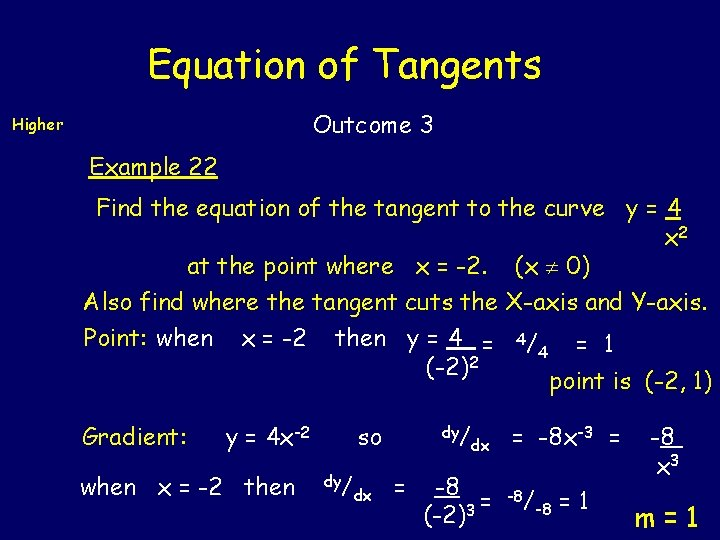Equation of Tangents Outcome 3 Higher Example 22 Find the equation of the tangent to the curve y = 4 x 2 at the point where x = -2. (x 0) Also find where the tangent cuts the X-axis and Y-axis. Point: when Gradient: x = -2 then y = 4 = (-2)2 y = 4 x-2 when x = -2 then so dy/ dx dy/ = dx -8 = (-2)3 4/ = 1 4 point is (-2, 1) = -8 x-3 = -8/ -8 =1 -8 x 3 m=1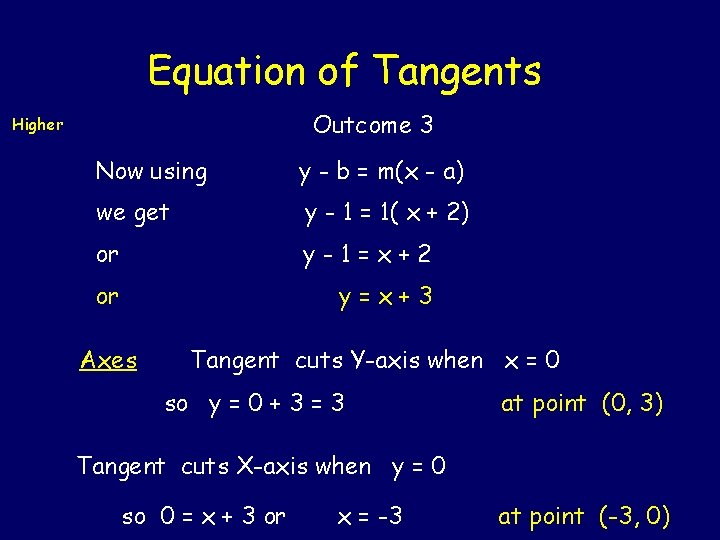Equation of Tangents Outcome 3 Higher Now using y - b = m(x - a) we get y - 1 = 1( x + 2) or y-1=x+2 or y=x+3 Axes Tangent cuts Y-axis when x = 0 so y = 0 + 3 = 3 at point (0, 3) Tangent cuts X-axis when y = 0 so 0 = x + 3 or x = -3 at point (-3, 0)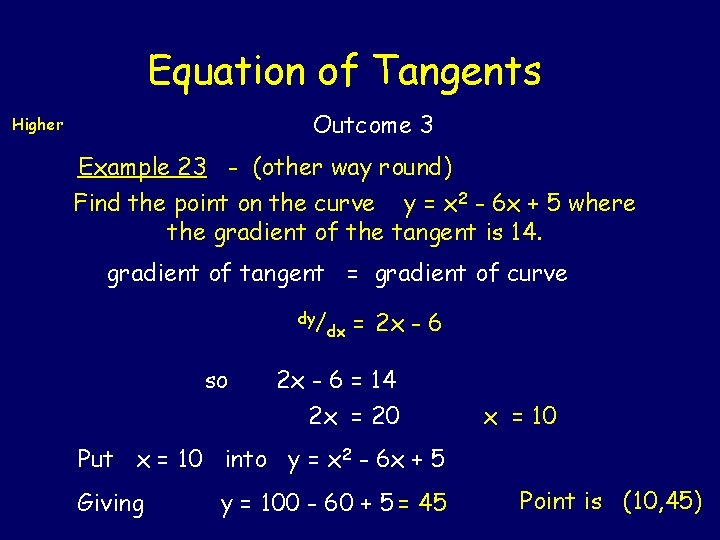Equation of Tangents Outcome 3 Higher Example 23 - (other way round) Find the point on the curve y = x 2 - 6 x + 5 where the gradient of the tangent is 14. gradient of tangent = gradient of curve dy/ so dx = 2 x - 6 = 14 2 x = 20 x = 10 Put x = 10 into y = x 2 - 6 x + 5 Giving y = 100 - 60 + 5 = 45 Point is (10, 45)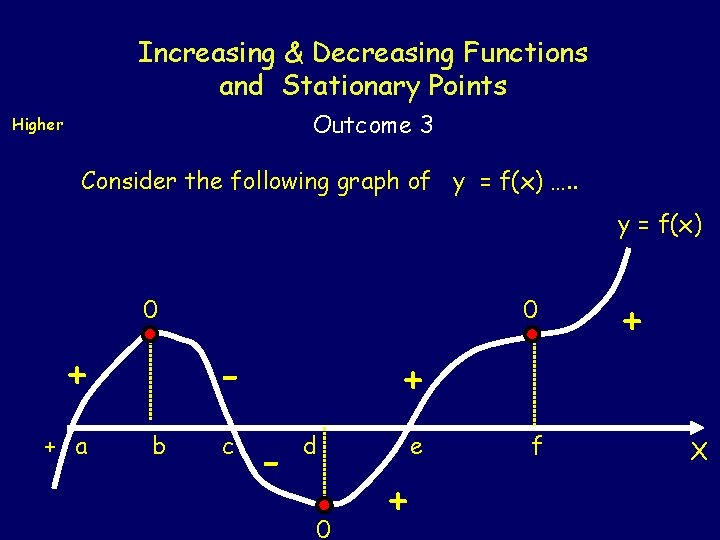Increasing & Decreasing Functions and Stationary Points Outcome 3 Higher Consider the following graph of y = f(x) …. . y = f(x) 0 + + a 0 b c + - d 0 e + f + X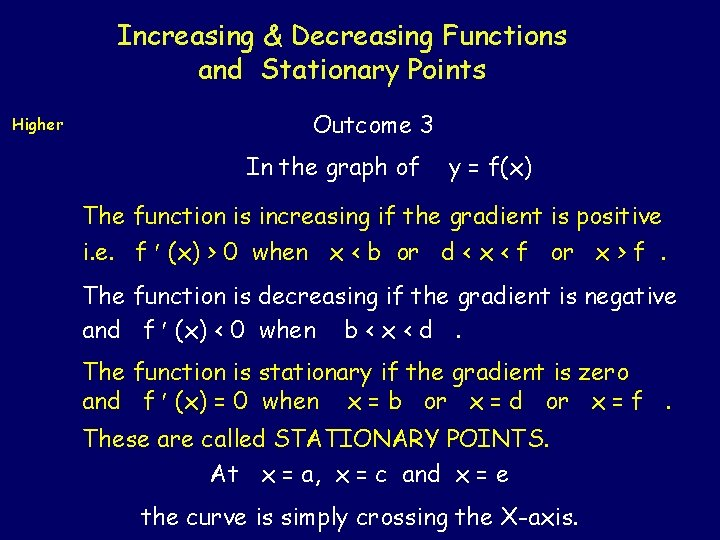Increasing & Decreasing Functions and Stationary Points Higher Outcome 3 In the graph of y = f(x) The function is increasing if the gradient is positive i. e. f (x) > 0 when x < b or d < x < f or x > f. The function is decreasing if the gradient is negative and f (x) < 0 when b < x < d. The function is stationary if the gradient is zero and f (x) = 0 when x = b or x = d or x = f. These are called STATIONARY POINTS. At x = a, x = c and x = e the curve is simply crossing the X-axis.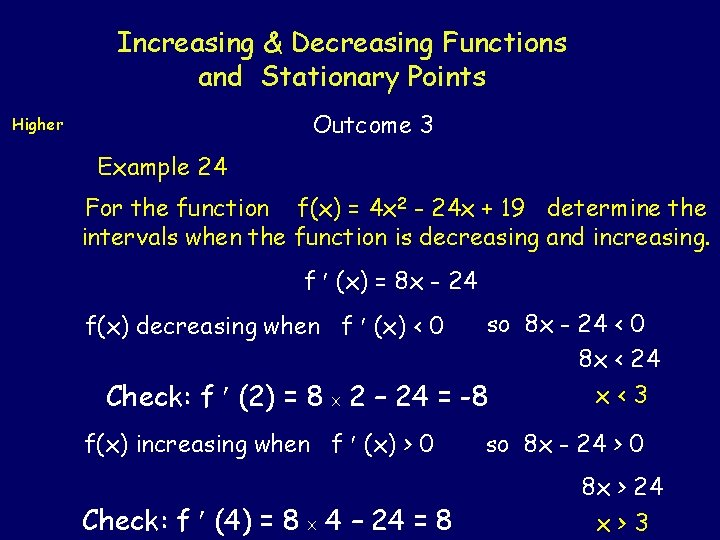Increasing & Decreasing Functions and Stationary Points Outcome 3 Higher Example 24 For the function f(x) = 4 x 2 - 24 x + 19 determine the intervals when the function is decreasing and increasing. f (x) = 8 x - 24 so 8 x - 24 < 0 8 x < 24 x<3 2 – 24 = -8 f(x) decreasing when f (x) < 0 Check: f (2) = 8 X f(x) increasing when f (x) > 0 Check: f (4) = 8 X 4 – 24 = 8 so 8 x - 24 > 0 8 x > 24 x>3Increasing & Decreasing Functions and Stationary Points Outcome 3 Higher Example 25 For the curve y = 6 x – 5/x 2 Determine if it is increasing or decreasing when x = 10. y = 6 x - 5 x-2 x 2 so dy/ dx = 6 + 10 x-3 = 6 + 10 x 3 when x = 10 Since dy/ dx = 6+ 10/ 1000 = 6. 01 > 0 then the function is increasing.Increasing & Decreasing Functions and Stationary Points Higher Outcome 3 Example 26 Show that the function g(x) = 1/3 x 3 -3 x 2 + 9 x -10 is never decreasing. g (x) = x 2 - 6 x + 9 = (x - 3)= (x - 3)2 Squaring a negative or a positive value produces a positive value, while 02 = 0. So you will never obtain a negative by squaring any real number. Since (x - 3)2 0 for all values of x then g (x) can never be negative so the function is never decreasing.Increasing & Decreasing Functions and Stationary Points Outcome 3 Higher Example 27 Determine the intervals when the function f(x) = 2 x 3 + 3 x 2 - 36 x + 41 is (a) Stationary (b) Increasing (c) Decreasing. f (x) = 6 x 2 + 6 x - 36 = 6(x 2 + x - 6) = 6(x + 3)(x - 2) Function is stationary when f (x) = 0 ie 6(x + 3)(x - 2) = 0 ie x = -3 or x=2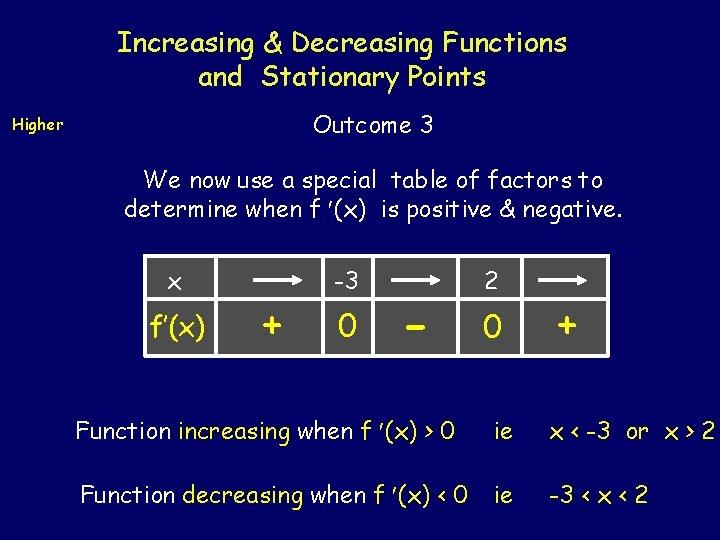Increasing & Decreasing Functions and Stationary Points Outcome 3 Higher We now use a special table of factors to determine when f (x) is positive & negative. x f’(x) + -3 0 - 2 0 + Function increasing when f (x) > 0 ie x < -3 or x > 2 Function decreasing when f (x) < 0 ie -3 < x < 2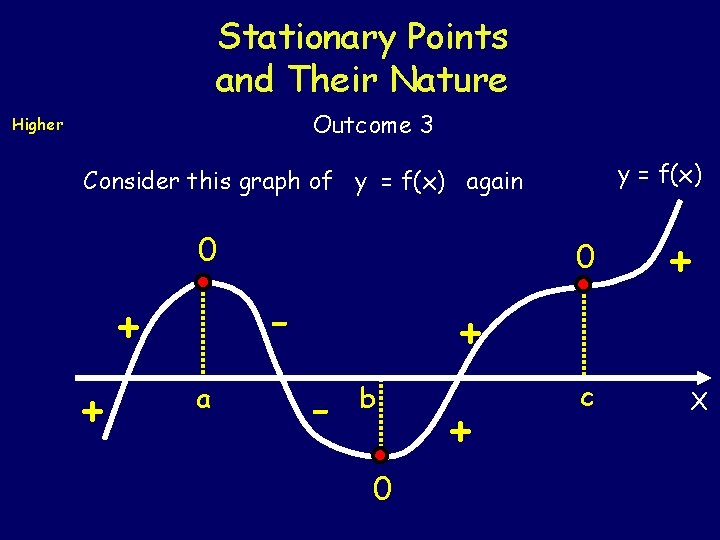Stationary Points and Their Nature Outcome 3 Higher y = f(x) Consider this graph of y = f(x) again 0 - + + 0 a + + - b 0 + c X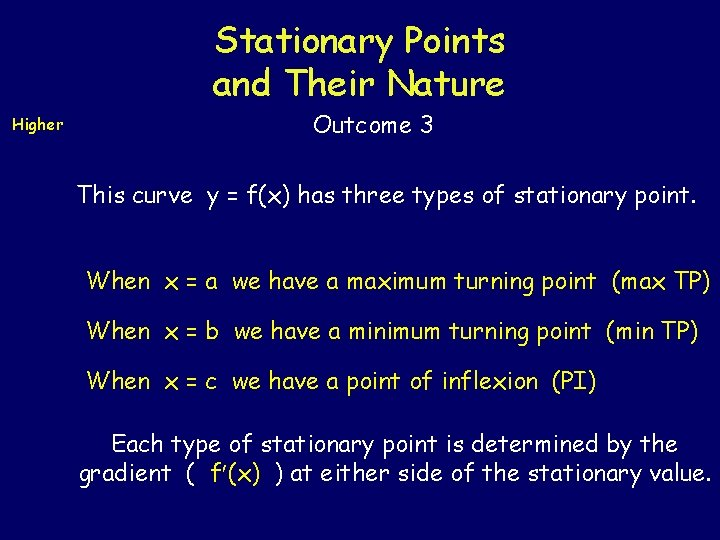Stationary Points and Their Nature Higher Outcome 3 This curve y = f(x) has three types of stationary point. When x = a we have a maximum turning point (max TP) When x = b we have a minimum turning point (min TP) When x = c we have a point of inflexion (PI) Each type of stationary point is determined by the gradient ( f (x) ) at either side of the stationary value.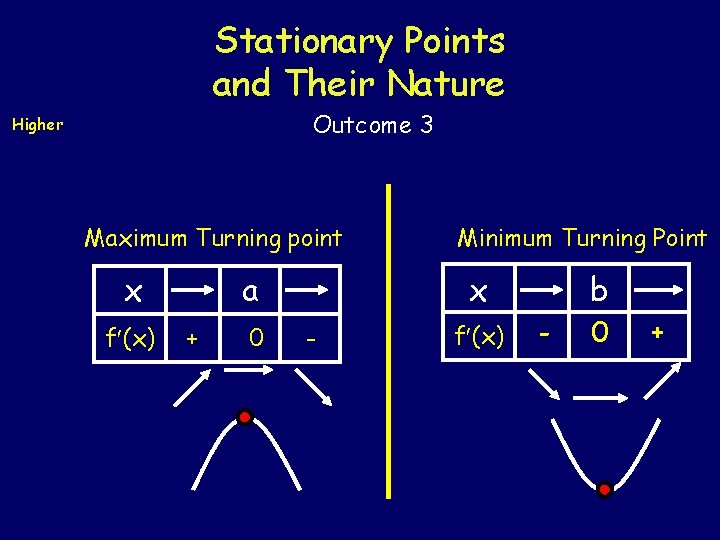Stationary Points and Their Nature Outcome 3 Higher Maximum Turning point x f (x) a + 0 Minimum Turning Point x - f (x) b - 0 +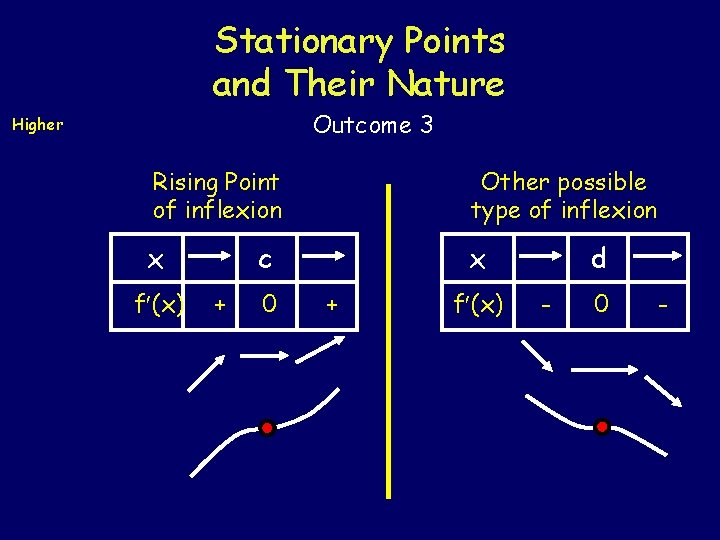Stationary Points and Their Nature Outcome 3 Higher Rising Point of inflexion Other possible type of inflexion x x f (x) c + 0 + f (x) d - 0 -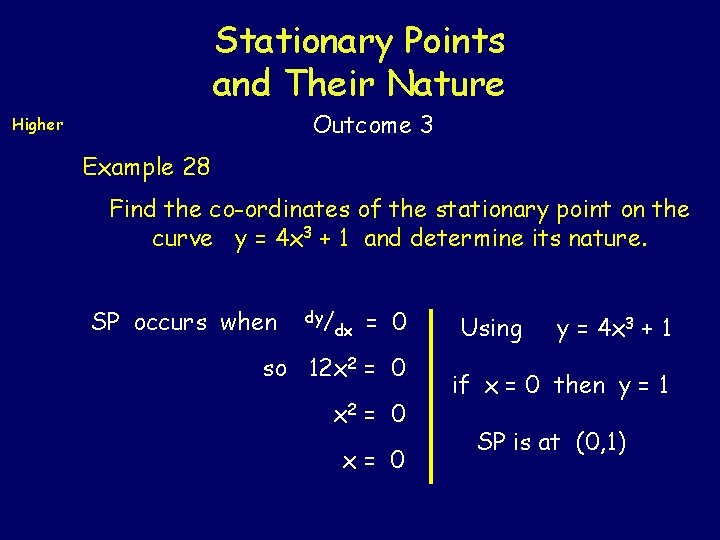Stationary Points and Their Nature Outcome 3 Higher Example 28 Find the co-ordinates of the stationary point on the curve y = 4 x 3 + 1 and determine its nature. SP occurs when dy/ dx = 0 so 12 x 2 = 0 x= 0 Using y = 4 x 3 + 1 if x = 0 then y = 1 SP is at (0, 1)Stationary Points and Their Nature Outcome 3 Higher Nature Table x dy/ dx 0 + dy/ dx = 12 x 2 So (0, 1) is a rising point of inflexion.Stationary Points and Their Nature Outcome 3 Higher Example 29 Find the co-ordinates of the stationary points on the curve y = 3 x 4 - 16 x 3 + 24 and determine their nature. SP occurs when dy/ dx = 0 So 12 x 3 - 48 x 2 = 0 12 x 2(x - 4) = 0 12 x 2 = 0 or (x - 4) = 0 x = 0 or x = 4 Using y = 3 x 4 - 16 x 3 + 24 if x = 0 then y = 24 if x = 4 then y = -232 SPs at (0, 24) & (4, -232)Stationary Points and Their Nature Outcome 3 Higher Nature Table x dy/ dx 4 0 - 0 + So (0, 24) is a Point of inflexion and (4, -232) is a minimum Turning Point dy/ dx=12 x 3 - 48 x 2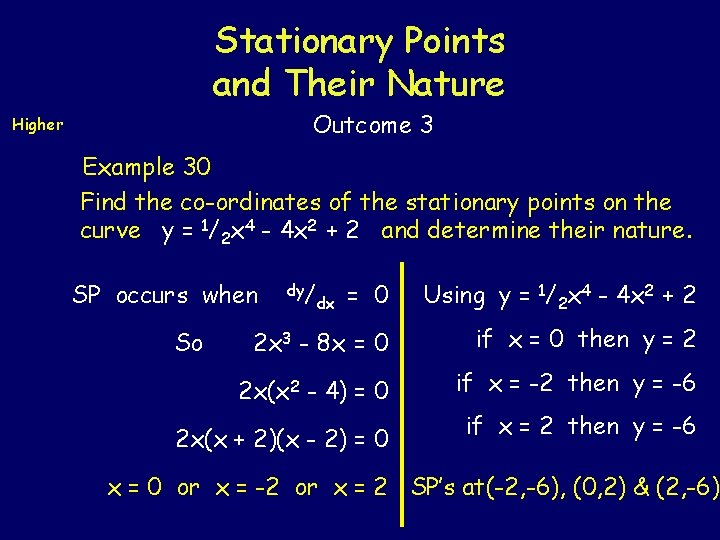Stationary Points and Their Nature Outcome 3 Higher Example 30 Find the co-ordinates of the stationary points on the curve y = 1/2 x 4 - 4 x 2 + 2 and determine their nature. = 0 Using y = 1/2 x 4 - 4 x 2 + 2 2 x 3 - 8 x = 0 if x = 0 then y = 2 2 x(x 2 - 4) = 0 if x = -2 then y = -6 SP occurs when So dy/ dx 2 x(x + 2)(x - 2) = 0 if x = 2 then y = -6 x = 0 or x = -2 or x = 2 SP’s at(-2, -6), (0, 2) & (2, -6)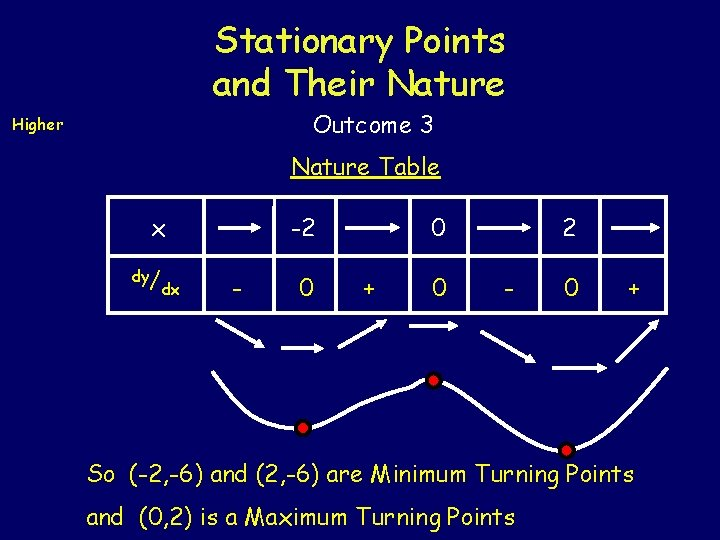Stationary Points and Their Nature Outcome 3 Higher Nature Table x dy/ dx -2 - 0 0 + 0 2 - 0 + So (-2, -6) and (2, -6) are Minimum Turning Points and (0, 2) is a Maximum Turning Points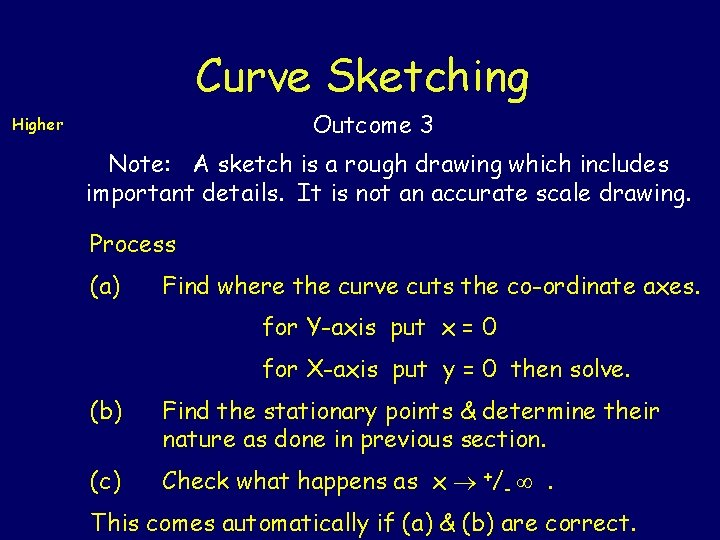Curve Sketching Outcome 3 Higher Note: A sketch is a rough drawing which includes important details. It is not an accurate scale drawing. Process (a) Find where the curve cuts the co-ordinate axes. for Y-axis put x = 0 for X-axis put y = 0 then solve. (b) Find the stationary points & determine their nature as done in previous section. (c) Check what happens as x +/- . This comes automatically if (a) & (b) are correct.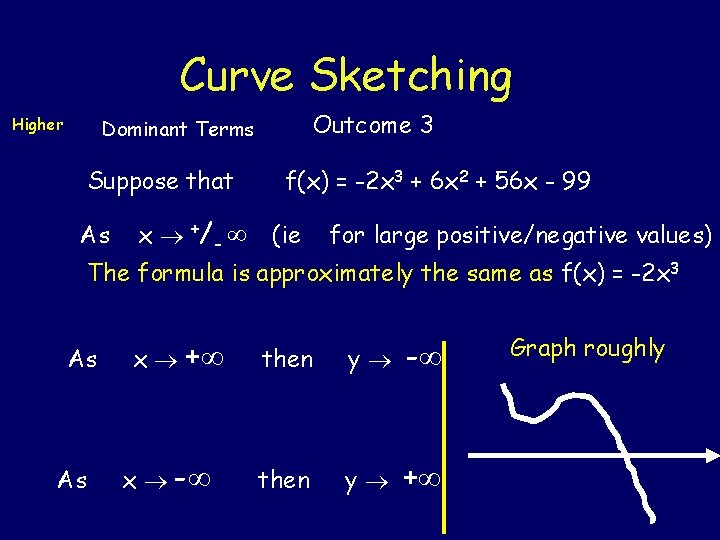Curve Sketching Higher Outcome 3 Dominant Terms Suppose that As x +/ - f(x) = -2 x 3 + 6 x 2 + 56 x - 99 (ie for large positive/negative values) The formula is approximately the same as f(x) = -2 x 3 As As x + x - then y + Graph roughlyCurve Sketching Outcome 3 Higher Example 31 Sketch the graph of y = -3 x 2 + 12 x + 15 (a) Axes If x = 0 then y = 15 If y = 0 then -3 x 2 + 12 x + 15 = 0 x 2 - 4 x - 5 = 0 (x + 1)(x - 5) = 0 x = -1 or x = 5 Graph cuts axes at (0, 15) , (-1, 0) and (5, 0) ( -3)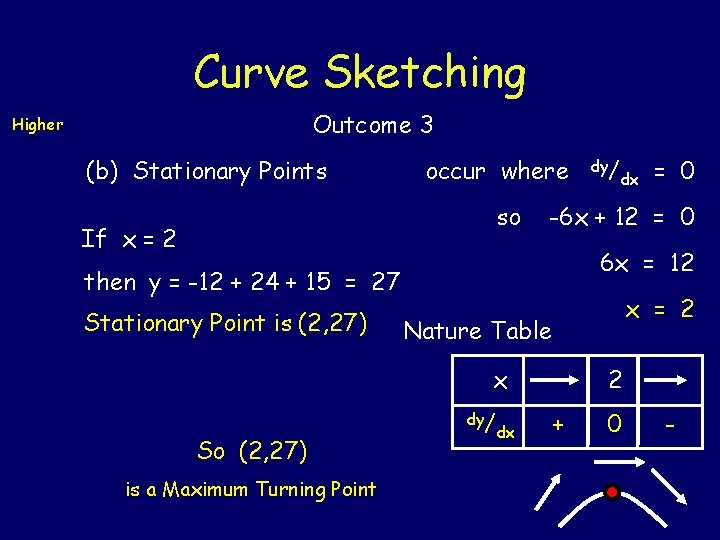Curve Sketching Outcome 3 Higher (b) Stationary Points occur where so If x = 2 is a Maximum Turning Point = 0 6 x = 12 x = 2 Nature Table x So (2, 27) dx -6 x + 12 = 0 then y = -12 + 24 + 15 = 27 Stationary Point is (2, 27) dy/ dx 2 + 0 -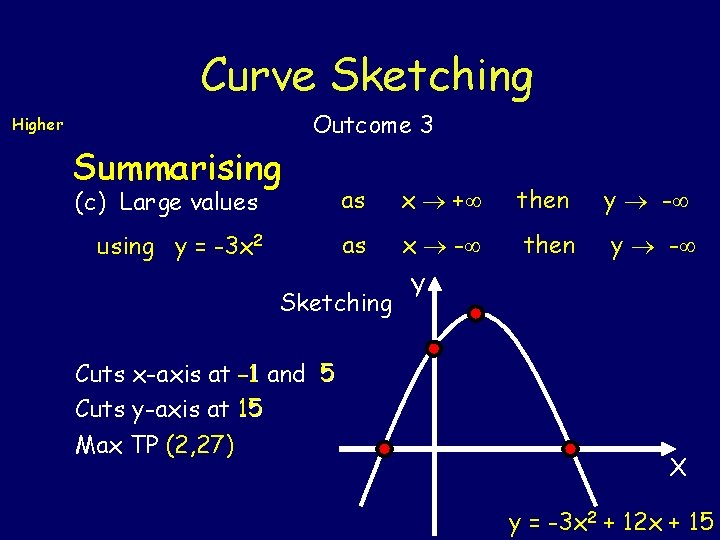Curve Sketching Higher Summarising Outcome 3 (c) Large values as x + then y - using y = -3 x 2 as x - then y - Sketching Y Cuts x-axis at -1 and 5 Cuts y-axis at 15 Max TP (2, 27) X y = -3 x 2 + 12 x + 15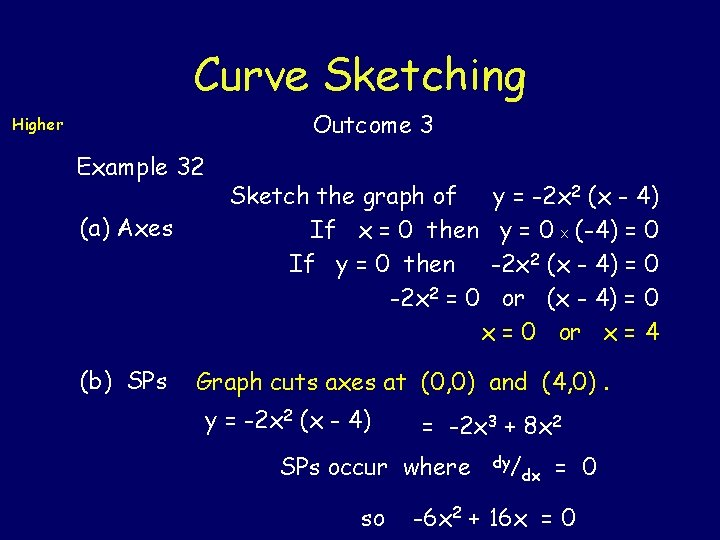Curve Sketching Outcome 3 Higher Example 32 (a) Axes (b) SPs Sketch the graph of If x = 0 then If y = 0 then -2 x 2 = 0 y = -2 x 2 (x - 4) y = 0 X (-4) = 0 -2 x 2 (x - 4) = 0 or (x - 4) = 0 x = 0 or x = 4 Graph cuts axes at (0, 0) and (4, 0). y = -2 x 2 (x - 4) = -2 x 3 + 8 x 2 SPs occur where so dy/ dx = 0 -6 x 2 + 16 x = 0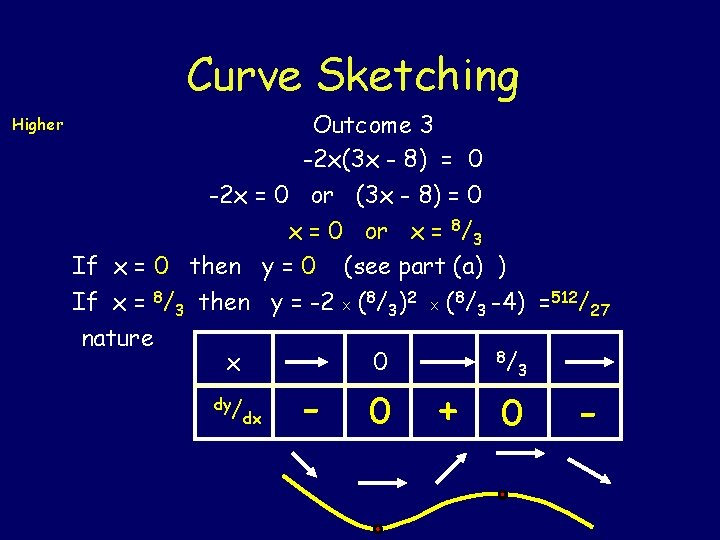Curve Sketching Higher Outcome 3 -2 x(3 x - 8) = 0 -2 x = 0 or (3 x - 8) = 0 x = 0 or x = 8/3 If x = 0 then y = 0 (see part (a) ) If x = 8/3 then y = -2 X (8/3)2 X (8/3 -4) =512/27 nature x dy/ dx - 0 0 8/ 3 + 0 -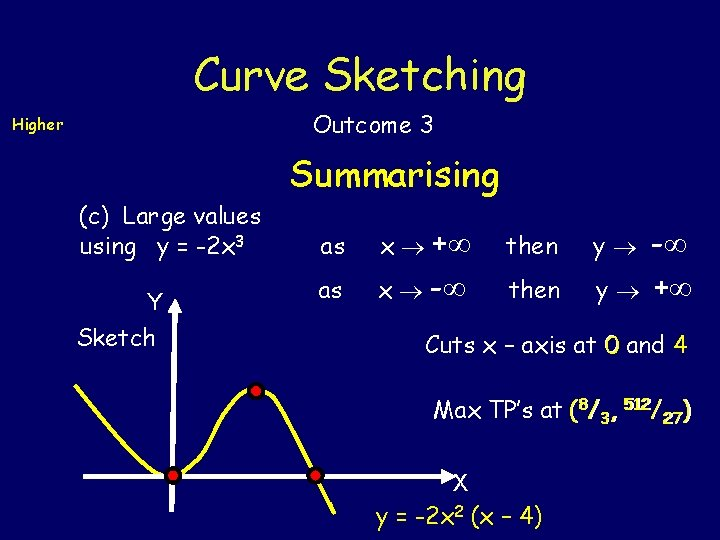Curve Sketching Outcome 3 Higher Summarising (c) Large values using y = -2 x 3 Y Sketch as as x + x - then y - + Cuts x – axis at 0 and 4 512/ ) Max TP’s at (88/33, 512 27 27 X y = -2 x 2 (x – 4)Curve Sketching Outcome 3 Higher Example 33 Sketch the graph of (a) Axes y = 8 + 2 x 2 - x 4 If x = 0 then y = 8 If y = 0 then 8 + 2 x 2 - x 4 = 0 Let u = x 2 so u 2 = x 4 (0, 8) Equation is now 8 + 2 u - u 2 = 0 (4 - u)(2 + u) = 0 (4 - x 2)(2 + x 2) = 0 or (2 + x) (2 - x)(2 + x 2) = 0 So x = -2 or x = 2 but x 2 -2 Graph cuts axes at (0, 8) , (-2, 0) and (2, 0)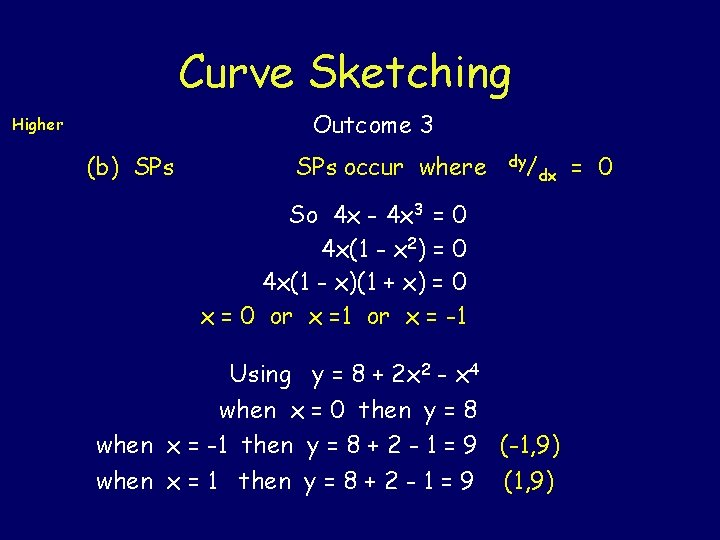Curve Sketching Outcome 3 Higher (b) SPs occur where dy/ dx So 4 x - 4 x 3 = 0 4 x(1 - x 2) = 0 4 x(1 - x)(1 + x) = 0 x = 0 or x =1 or x = -1 Using y = 8 + 2 x 2 - x 4 when x = 0 then y = 8 when x = -1 then y = 8 + 2 - 1 = 9 (-1, 9) when x = 1 then y = 8 + 2 - 1 = 9 (1, 9) = 0Curve Sketching Outcome 3 Higher nature x dy/ dx -1 0 1 + 0 - 0 + 0 - So (0, 8) is a min TP while (-1, 9) & (1, 9) are max TPs.Curve Sketching Higher Summarising Sketch is Y Outcome 3 (c) Large values Using y = - x 4 as as x + x - then y - - Cuts x – axis at -2 and 2 Cuts y – axis at 8 Max TP’s at (-1, 9) (1, 9) X y = 8 + 2 x 2 - x 4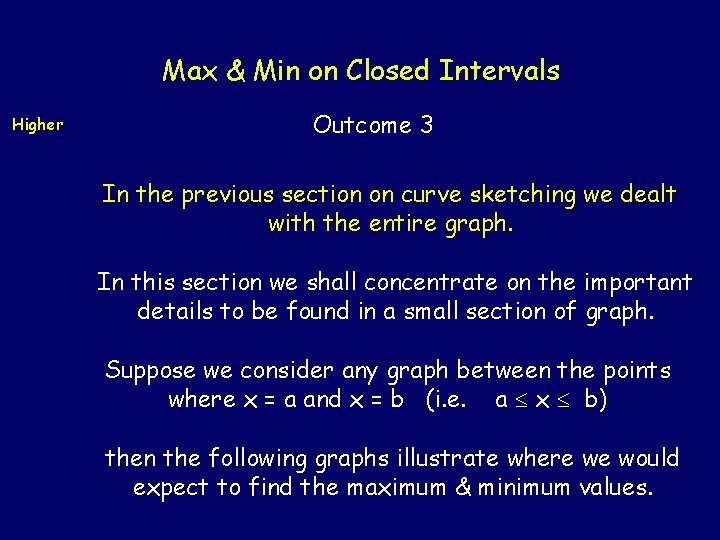Max & Min on Closed Intervals Higher Outcome 3 In the previous section on curve sketching we dealt with the entire graph. In this section we shall concentrate on the important details to be found in a small section of graph. Suppose we consider any graph between the points where x = a and x = b (i. e. a x b) then the following graphs illustrate where we would expect to find the maximum & minimum values.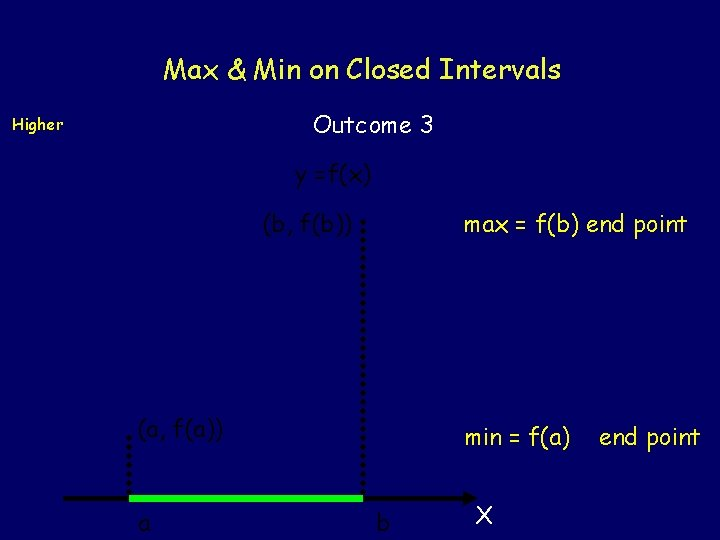Max & Min on Closed Intervals Outcome 3 Higher y =f(x) (b, f(b)) max = f(b) end point (a, f(a)) a min = f(a) b X end point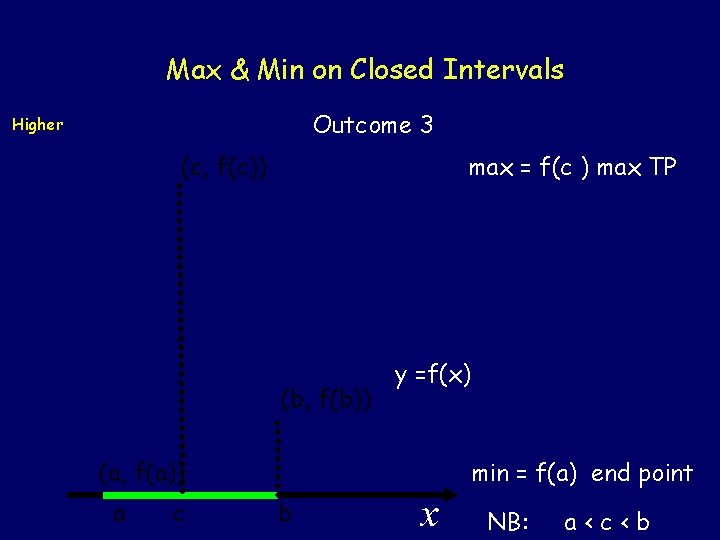Max & Min on Closed Intervals Outcome 3 Higher (c, f(c)) max = f(c ) max TP (b, f(b)) y =f(x) min = f(a) end point (a, f(a)) a c b x NB: a<c<bMax & Min on Closed Intervals Outcome 3 y =f(x) max = f(b) end point Higher (b, f(b)) (a, f(a)) (c, f(c)) a c b min = f(c) min TP x NB: a<c<bMax & Min on Closed Intervals Outcome 3 Higher From the previous three diagrams we should be able to see that the maximum and minimum values of f(x) on the closed interval a x b can be found either at the end points or at a stationary point between the two end points Example 34 Find the max & min values of y = 2 x 3 - 9 x 2 in the interval where -1 x 2. End points If x = -1 then y = -2 - 9 = -11 If x = 2 then y = 16 - 36 = -20Max & Min on Closed Intervals Outcome 3 Higher Stationary points dy/ dx = 6 x 2 - 18 x = 6 x(x - 3) SPs occur where dy/dx = 0 6 x(x - 3) = 0 6 x = 0 or x - 3 = 0 x = 0 or x = 3 not in interval If x = 0 then y = 0 - 0 = 0 Hence for -1 x 2 , max = 0 & min = -20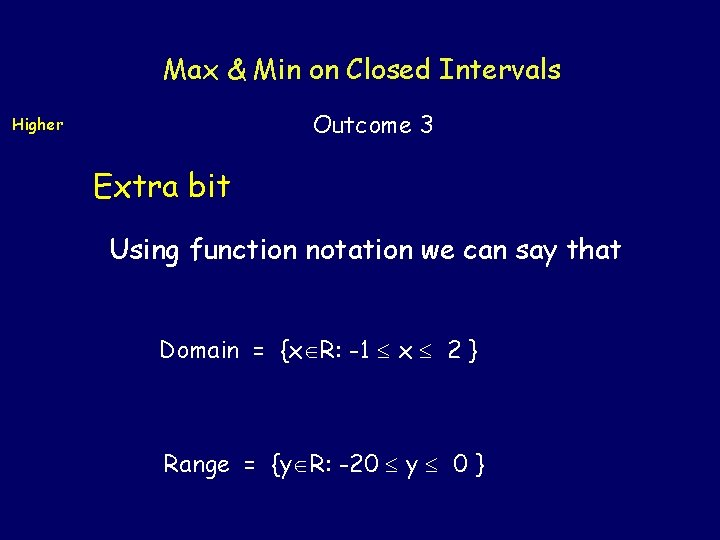Max & Min on Closed Intervals Outcome 3 Higher Extra bit Using function notation we can say that Domain = {x R: -1 x 2 } Range = {y R: -20 y 0 }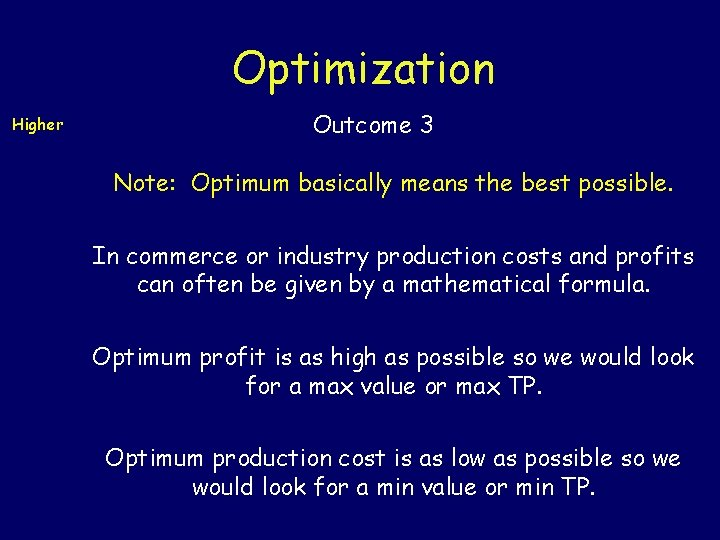Optimization Higher Outcome 3 Note: Optimum basically means the best possible. In commerce or industry production costs and profits can often be given by a mathematical formula. Optimum profit is as high as possible so we would look for a max value or max TP. Optimum production cost is as low as possible so we would look for a min value or min TP.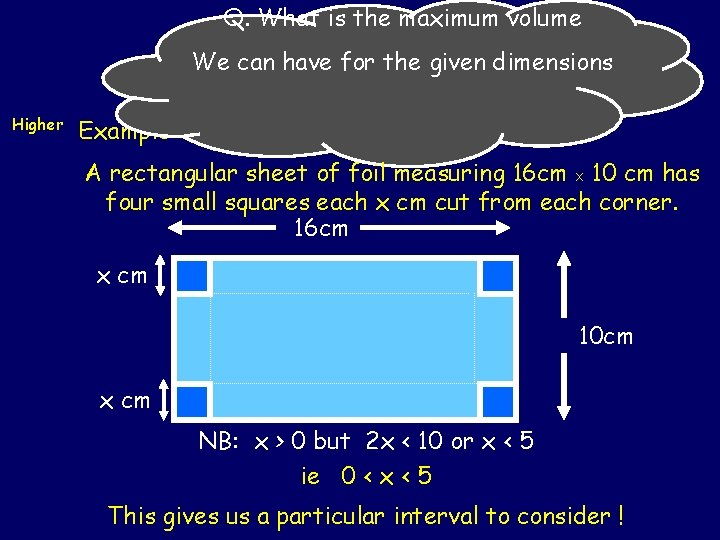Q. What is the maximum volume We can have for the given dimensions Optimization Higher Example 35 Outcome 3 A rectangular sheet of foil measuring 16 cm X 10 cm has four small squares each x cm cut from each corner. 16 cm x cm 10 cm x cm NB: x > 0 but 2 x < 10 or x < 5 ie 0 < x < 5 This gives us a particular interval to consider !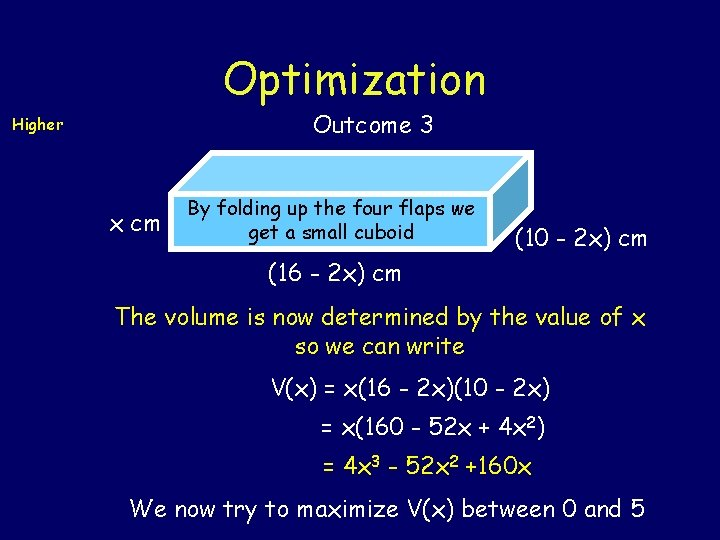Optimization Outcome 3 Higher x cm By folding up the four flaps we get a small cuboid (10 - 2 x) cm (16 - 2 x) cm The volume is now determined by the value of x so we can write V(x) = x(16 - 2 x)(10 - 2 x) = x(160 - 52 x + 4 x 2) = 4 x 3 - 52 x 2 +160 x We now try to maximize V(x) between 0 and 5Optimization Outcome 3 Higher End Points Considering the interval 0 < x < 5 V(0) = 0 X 16 X 10 = 0 V(5) = 5 X 6 X 0 = 0 SPs V '(x) = 12 x 2 - 104 x + 160 = 4(3 x 2 - 26 x + 40) = 4(3 x - 20)(x - 2)Optimization Outcome 3 Higher SPs occur when V '(x) = 0 ie 4(3 x - 20)(x - 2) = 0 3 x - 20 = 0 or x - 2 = 0 ie x = 20/ 3 or x = 2 not in interval When x = 2 then in interval V(2) = 2 X 12 X 6 = 144 We now check gradient near x = 2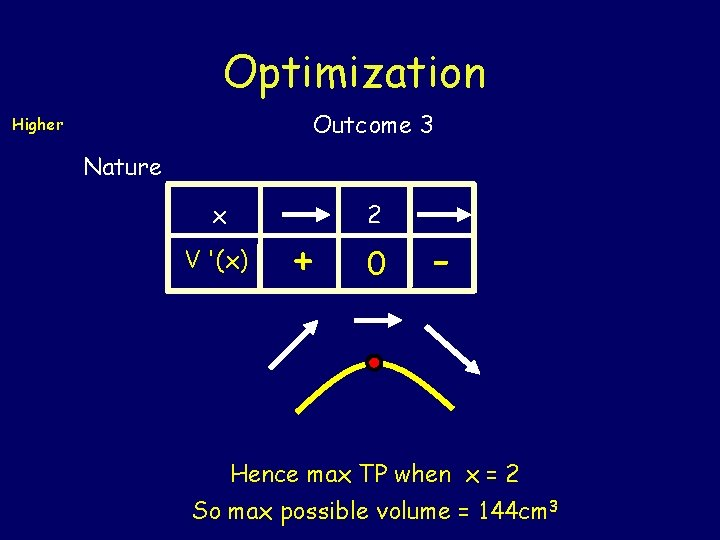Optimization Outcome 3 Higher Nature x V '(x) + 2 0 - Hence max TP when x = 2 So max possible volume = 144 cm 3Optimization Higher Example 36 Outcome 3 When a company launches a new product its share of the market after x months is calculated by the formula (x 2) So after 5 months the share is S(5) = 2/5 – 4/25 = 6/25 Find the maximum share of the market that the company can achieve.Optimization Outcome 3 Higher End points S(2) = 1 – 1 = 0 There is no upper limit but as x S(x) 0. SPs occur where S (x) = 0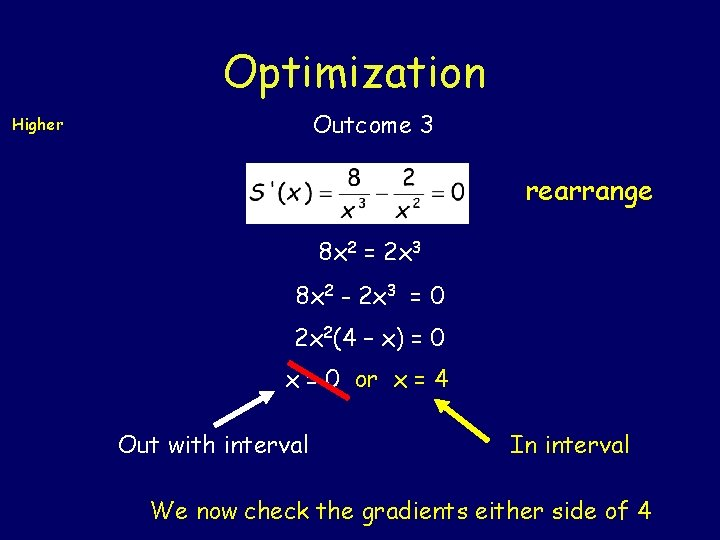Optimization Outcome 3 Higher rearrange 8 x 2 = 2 x 3 8 x 2 - 2 x 3 = 0 2 x 2(4 – x) = 0 x = 0 or x = 4 Out with interval In interval We now check the gradients either side of 4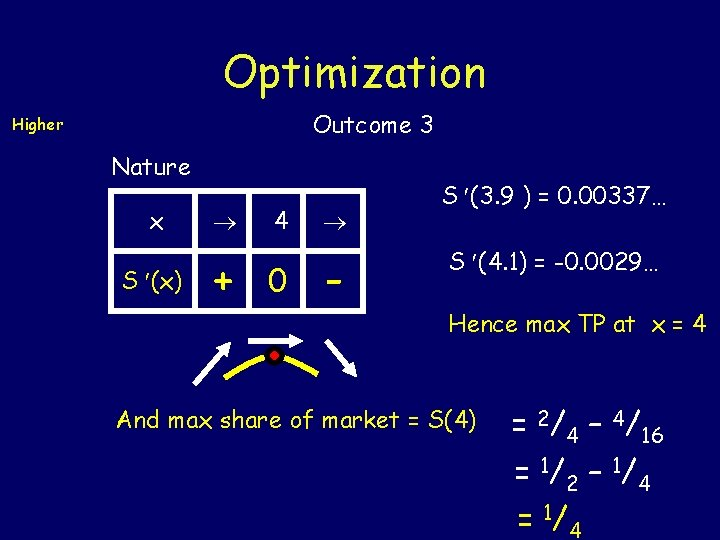Optimization Outcome 3 Higher Nature x S (x) + 4 0 - S (3. 9 ) = 0. 00337… S (4. 1) = -0. 0029… Hence max TP at x = 4 And max share of market = S(4) = 2/4 – 4/16 = 1/ 2 – 1/ 4 = 1/ 4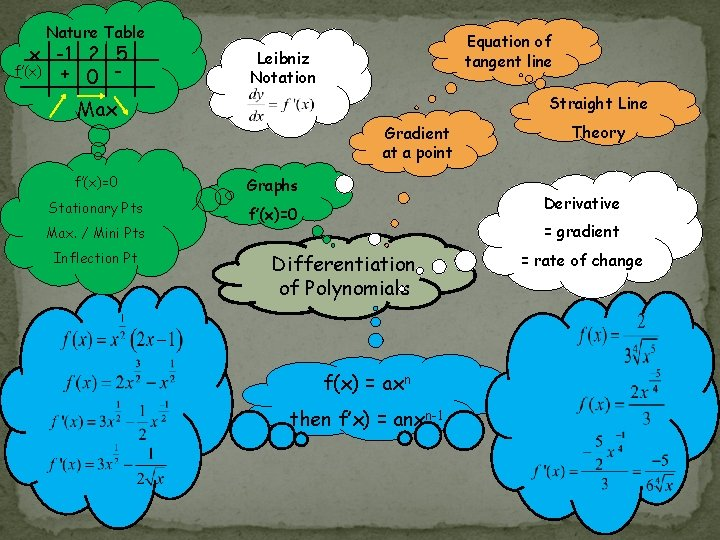Nature Table x -1 2 5 + 0 - f’(x) Leibniz Notation Straight Line Max Gradient at a point f’(x)=0 Graphs Stationary Pts f’(x)=0 Max. / Mini Pts Inflection Pt Equation of tangent line Theory Derivative = gradient Differentiation of Polynomials f(x) = axn then f’x) = anxn-1 = rate of change